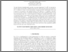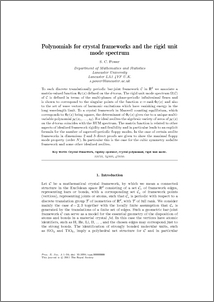Polynomials for crystal frameworks and the rigid unit mode spectrum

Power, Stephen (2014) Polynomials for crystal frameworks and the rigid unit mode spectrum. Philosophical Transactions of the Royal Society A: Mathematical, Physical & Engineering Sciences, 372 (2008). ISSN 1471-2962Preview
PDF (PowerPhilTranRevised)
PowerPhilTranRevised.pdf - Accepted Version

Abstract

To each discrete translationally periodic bar-joint framework $\C$ in $\bR^d$ we associate a matrix-valued function $\Phi_\C(z)$ defined on the $d$-torus. The rigid unit mode spectrum $\Omega(\C)$ of $\C$ is defined in terms of the multi-phases of phase-periodic infinitesimal flexes and is shown to correspond to the singular points of the function $z \to \rank \Phi_\C(z)$ and also to the set of wave vectors of harmonic excitations which have vanishing energy in the long wavelength limit. To a crystal framework in Maxwell counting equilibrium the determinant of $\Phi_\C(z)$ is defined and gives rise to a unique multi-variable polynomial $p_\C(z_1,\dots ,z_d)$. For ideal zeolites the algebraic variety of zeros of $p_\C(z)$ on the $d$-torus coincides with the RUM spectrum. The matrix function is related to other aspects of idealised framework rigidity and flexibility and in particular leads to an explicit formula for the number of supercell-periodic floppy modes. In the case of certain zeolite frameworks in dimensions $2$ and $3$ direct proofs are given to show the maximal floppy mode property (order $N$). In particular this is the case for the cubic symmetry sodalite framework and other idealised zeolites.

Item Type:
Journal Article
Journal or Publication Title:
Philosophical Transactions of the Royal Society A: Mathematical, Physical & Engineering Sciences
Uncontrolled Keywords:
/dk/atira/pure/researchoutput/libraryofcongress/qa
Subjects:
Departments:
ID Code:
64355
Deposited By:
Deposited On:
08 May 2013 10:14
Refereed?:
Yes
Published?:
Published